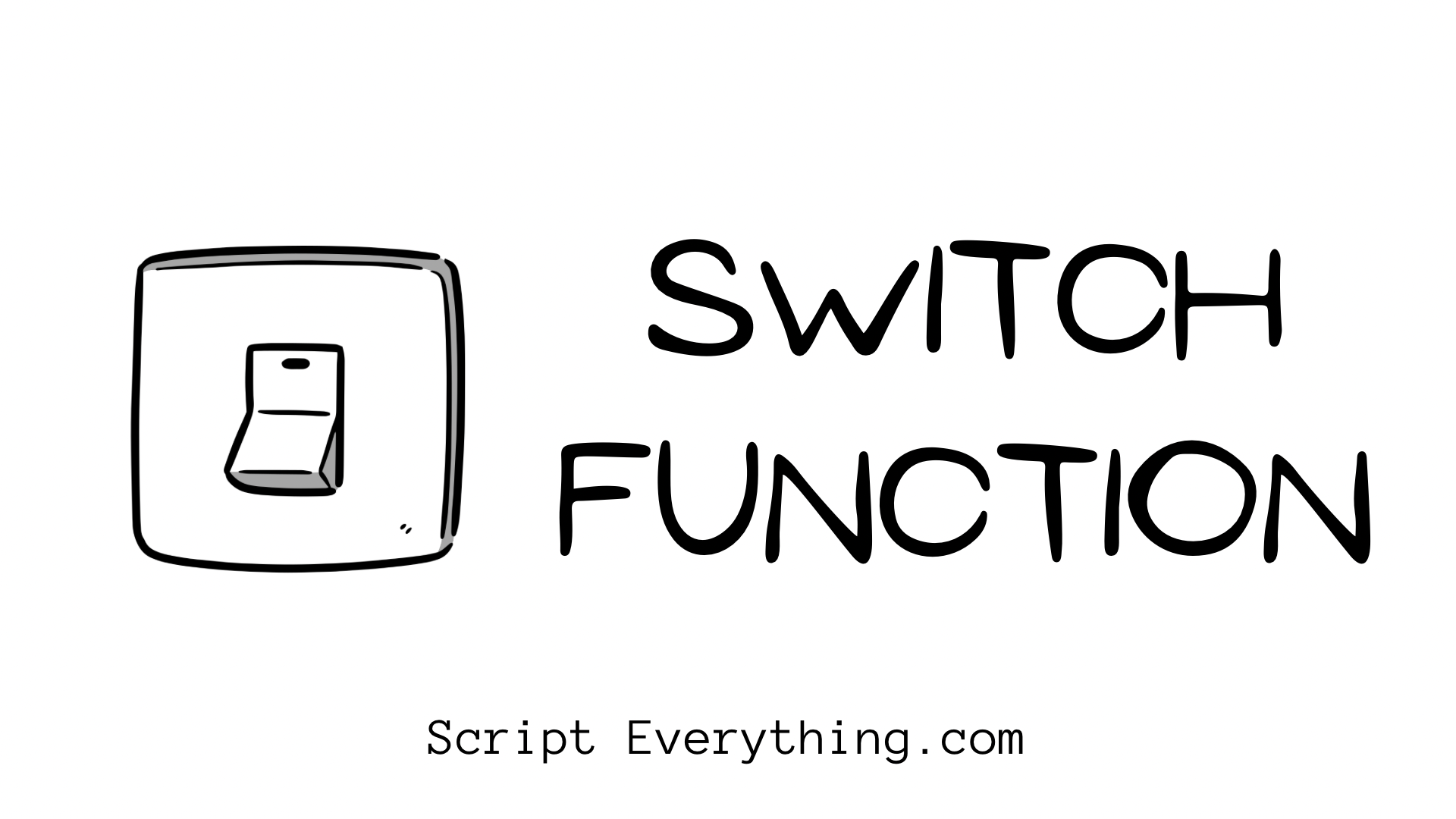# How To Use The SWITCH Function In Google Sheets?The ``` SWITCH() ``` function in Google Sheets is handy when dealing with multiple criteria based on a single result.

The ``` SWITCH() ``` function takes at least 3 parameters, with the first parameter being the value to evaluate, the second and third parameter representing a pair of combined cases and values. There’s also an optional final parameter that acts as the default result if no cases are satisfied.

Here is a simple demonstration of the SWITCH function:

As demonstrated above the ``` SWITCH ``` function in its first parameter contains the ``` WEEKDAY ``` function which takes 2 values: a reference to a date cell ``` A1 ``` and the type of result sought (with ``` 1 ``` being the default type if no value is entered and therefore 1 representing Sunday as 1, Monday as 2, etc).

Depending on the day of the week the ``` SWITCH ``` function then proceeds with evaluating each case statement. The first case statement handles what to do if the result is ``` 1 ``` and if this matches to return the value ``` "SUN" ``` . As the value of the ``` WEEKDAY ``` function will return ``` 4 ``` it continues to progress through each case statement until it matches.

With this example, all cases have a corresponding value representing an abbreviated day of the week. If I wanted to change the formula so that ``` SAT ``` and ``` SUN ``` just reported ``` WEEKEND ``` I could change the formula to this instead:

As you can see from the above example with 18th December 2021 representing a weekend day, no cases are matched to the result of the first parameter and therefore the default (the final parameter) is returned.

## Why Not Use ``` IFS ``` Instead?

The main differences between the ``` SWITCH ``` function and the ``` IFS ``` function are that the ``` IFS ``` function can evaluate multiple criteria, and it does not provide a default value if NO condition is met, therefore you would need to ensure your formula captures all possible scenarios.

The alternative formula using the ``` IFS ``` formula for the above example would be something like this:

``=IFS(WEEKDAY(A1,2)=1,"MON",WEEKDAY(A1,2)=2,"TUE",WEEKDAY(A1,2)=3,"WED",WEEKDAY(A1,2)=4,"THU",WEEKDAY(A1,2)=5,"FRI",WEEKDAY(A1,2)>5,"WEEKEND")``

As you can see from the above formula it’s very long considering the alternative ``` SWITCH ``` formula! As each condition needs to be evaluated it all would need to contain the ``` WEEKDAY ``` formula to determine the correct value. I changed the second parameter to 2 to make it easier to capture the weekends as Saturday and Sunday would evaluate to greater than 5.

The ``` SWITCH ``` function in comparison evaluates the same expression and only evaluates the result according to each case statement.

## Can A Case Statement Be An Expression?

Unfortunately, the ``` SWITCH ``` function is quite strict on the case statements inside and cannot contain an expression. The case parameter must match a value likely to be returned from the first parameter’s expression.

For example, the following function would produce an error:

``=SWITCH(WEEKDAY(A1,2),1,"MON",2,"TUE",3,"WED",4,"THU",5,"FRI",">5","WEEKEND")``

Using an expression does not work and will just produce an ``` #N/A ``` result as the case needs to match the result from the first parameter.

If you want to see another example of the ``` SWITCH ``` function check my other post where I reduced my formula code by 20% .

## Summary

The ``` SWITCH ``` function provides an easy way to provide a result based on a single expression. It can be more succinct than the corresponding ``` IFS ``` function, however, does have the drawback that the case statements cannot be expressions themselves and must match actual results.

Next, you might like to explore more about the ``` IFS ``` formula .

Author of scripteverything.com, Ryan has been dabbling in code since the late '90s when he cut his teeth by exploring VBA in Excel when trying to do something more. Having his eyes opened with the potential of automating repetitive tasks, he expanded to Python and then moved over to scripting languages such as HTML, CSS, Javascript and PHP. When he is not behind a screen, Ryan enjoys a good bush walk with the family during the cooler months, and going with them to the beach during the warmer months.